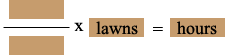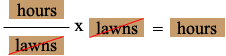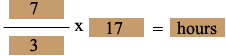SEARCH HOMEMath Central Quandaries & QueriesQuestion from kevin, a student: I need help setting up a equation to solve the following question: If a gardener can mow 3 lawns in 7 hours, how long should it take him to mow 17 lawns. I can solve the problem, but I don't know how to set up the equation.Hi Kevin,

This is a rate problem and I know I have to use the rate at which the gardner mows the lawn but I don't know whether to express the rate as lawns per hour or hours per lawn. I let the units tell me what to do.

I want an answer in hours when he mows 17 lawns so my equation is going to look like

rate times number of lawns equals number of hours, orThus, in order for the units to cancel I need the rate in hours per lawnThis is then my equation which yieldsI hope this helps,
PennyMath Central is supported by the University of Regina and The Pacific Institute for the Mathematical Sciences.